Minima/ maxima (statement questions) - Geometry questions

Chapter 6 Class 12 Application of Derivatives
Concept wise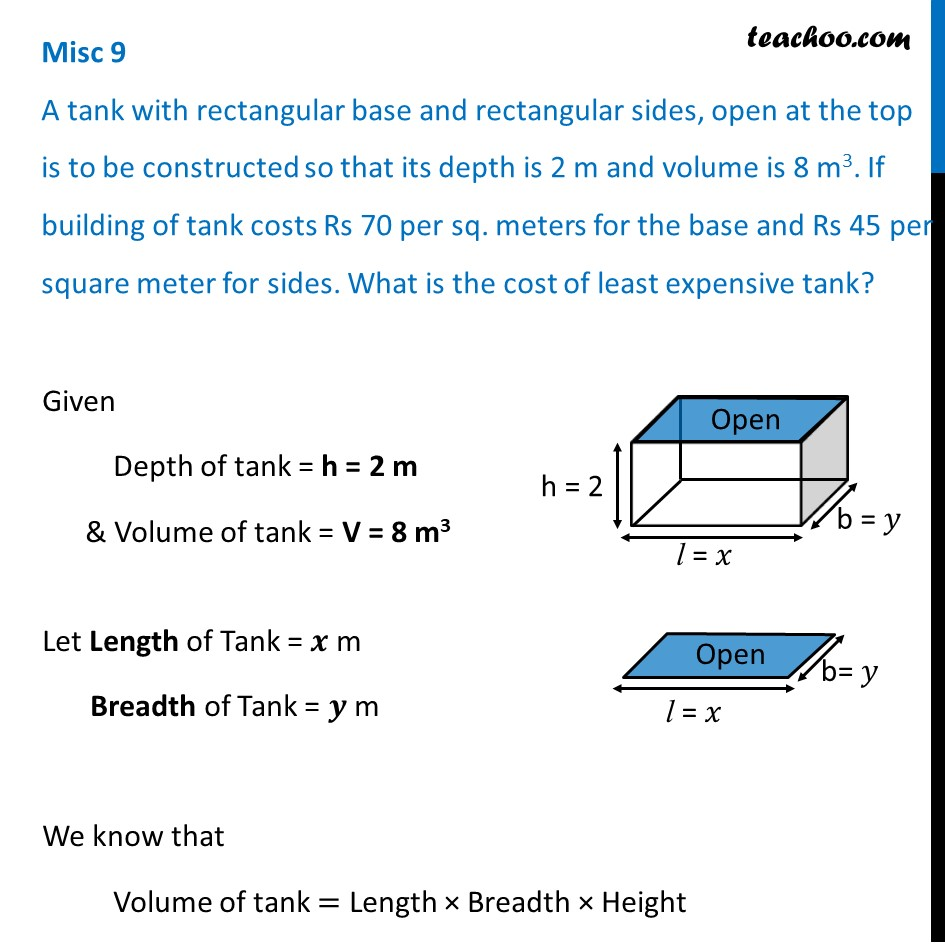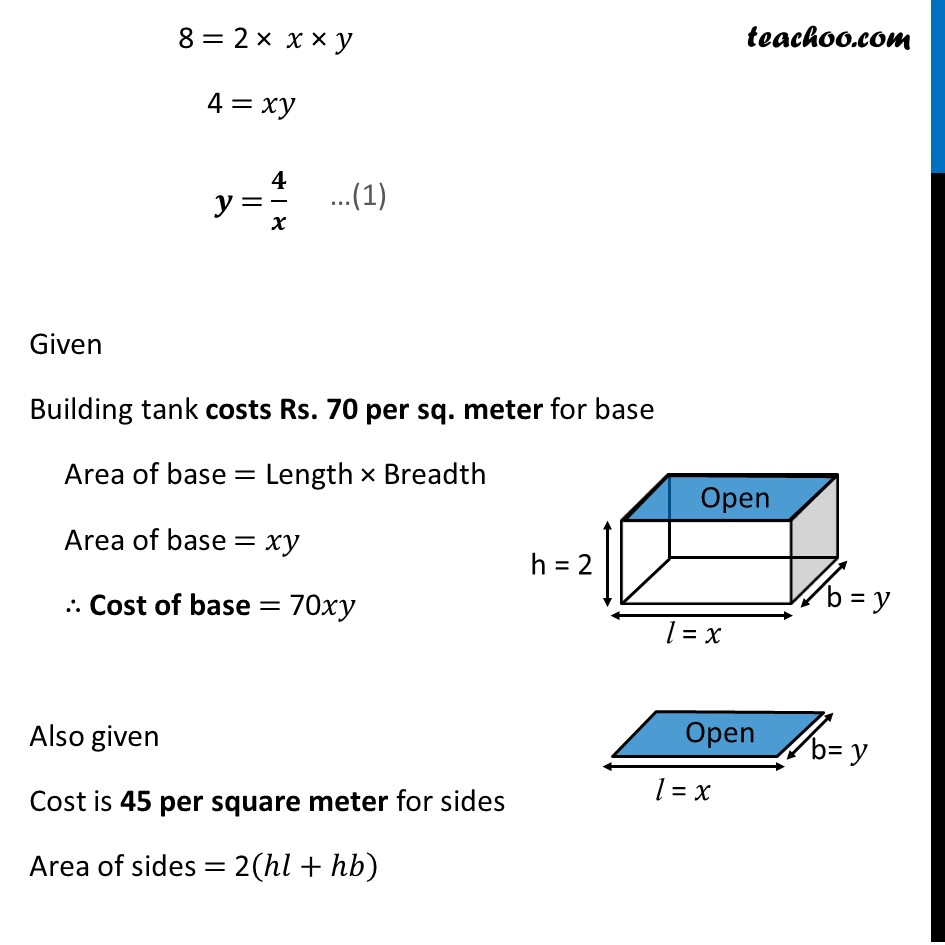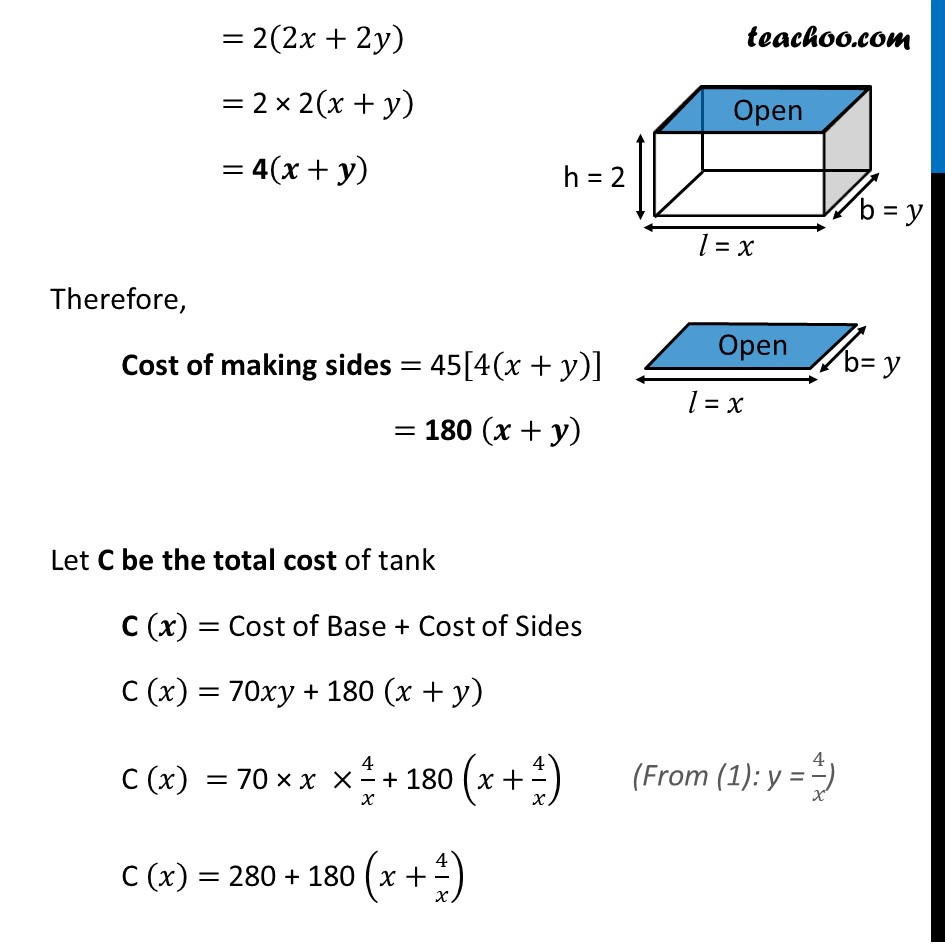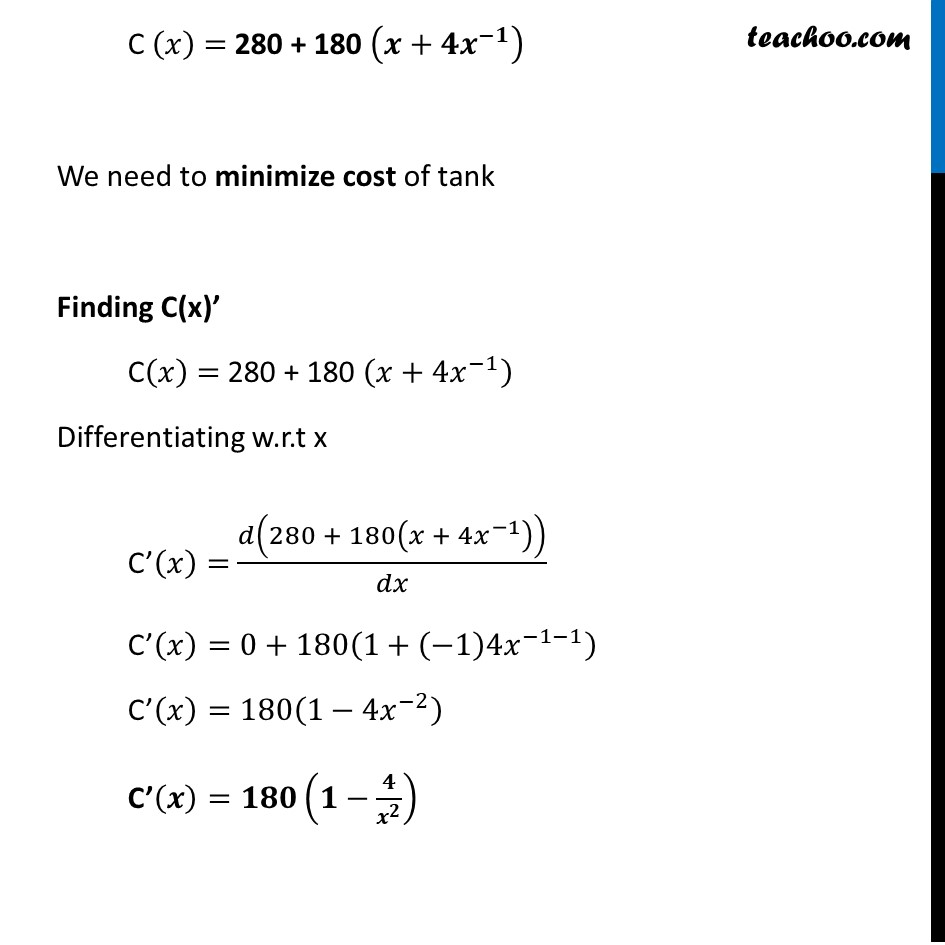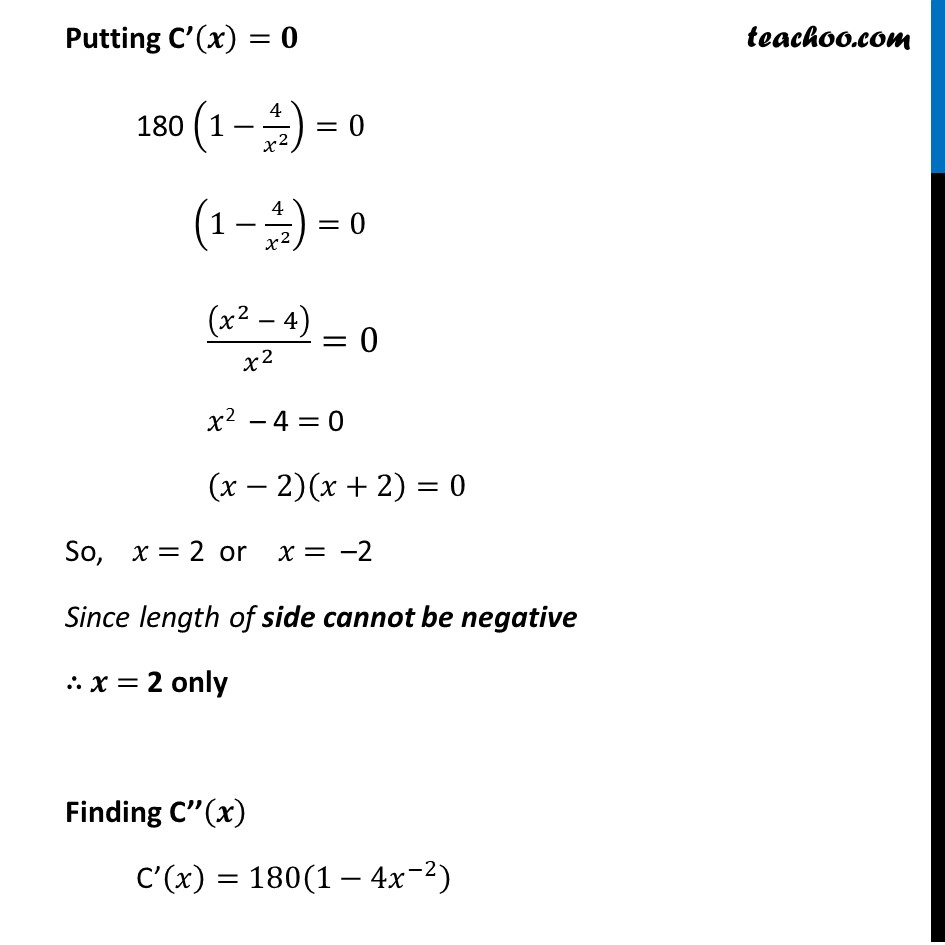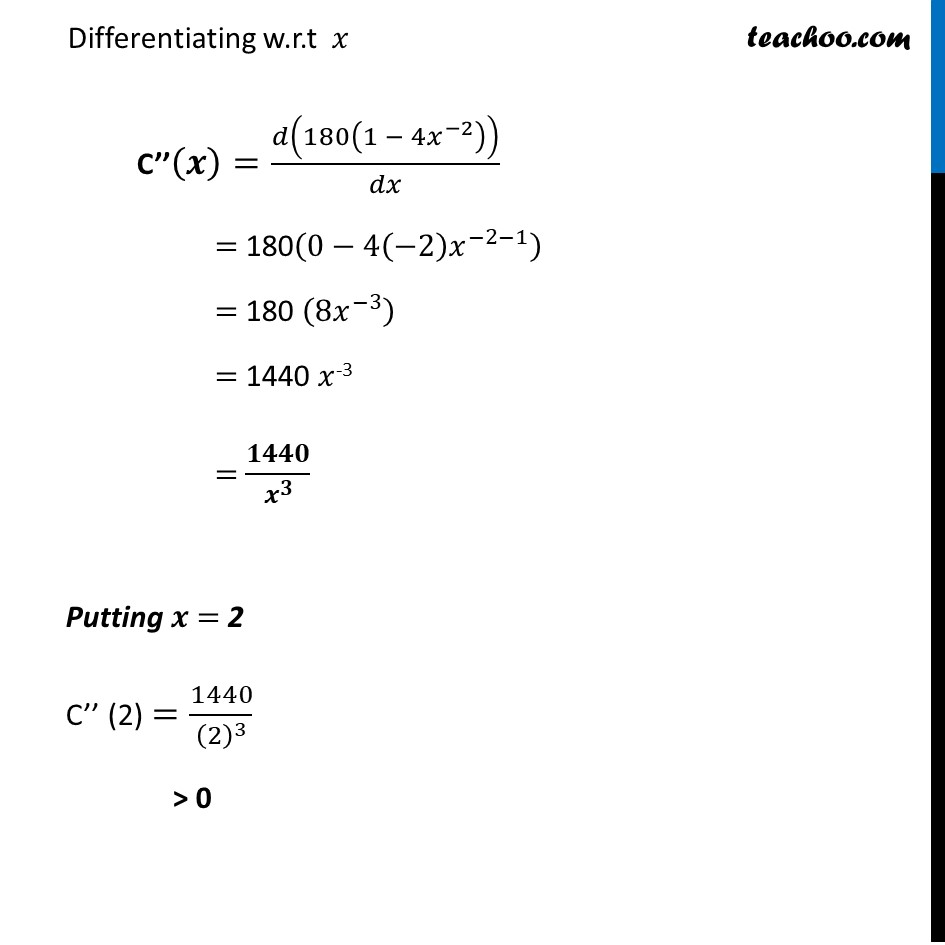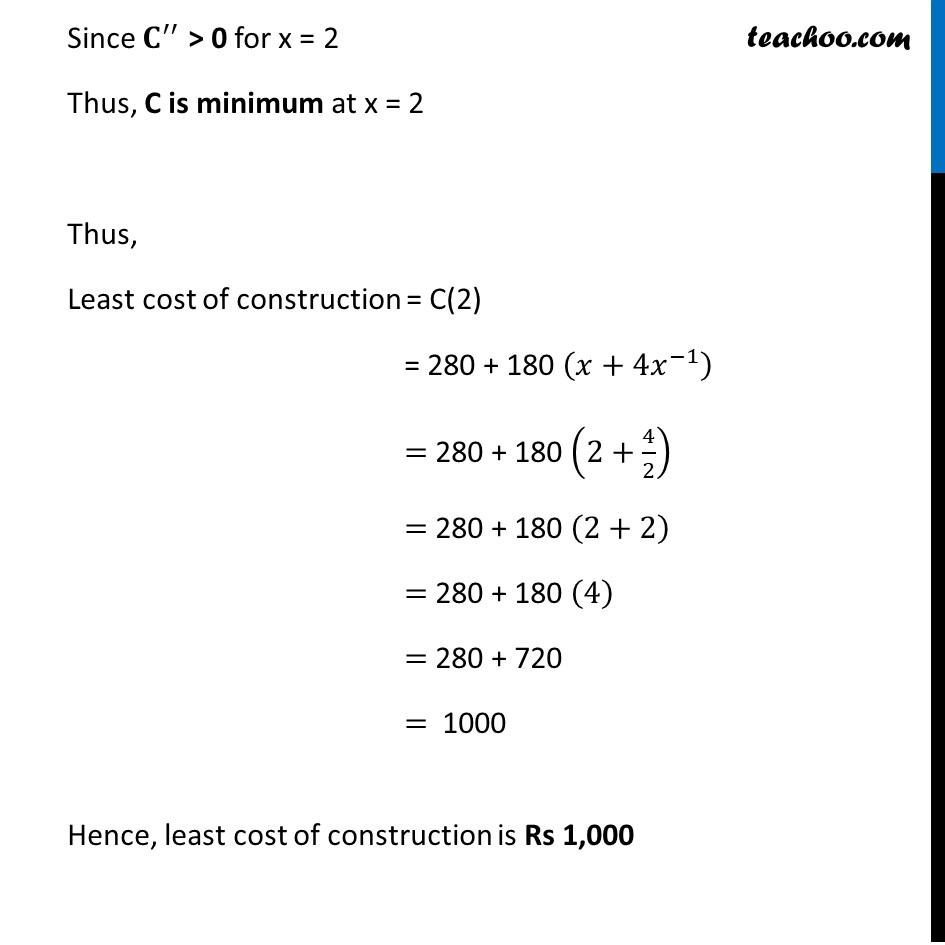This video is only available for Teachoo black users

Introducing your new favourite teacher - Teachoo Black, at only ₹83 per month

### Transcript

Misc 9 A tank with rectangular base and rectangular sides, open at the top is to be constructed so that its depth is 2 m and volume is 8 m3. If building of tank costs Rs 70 per sq. meters for the base and Rs 45 per square meter for sides. What is the cost of least expensive tank?Given Depth of tank = h = 2 m & Volume of tank = V = 8 m3 Let Length of Tank = 𝒙 m Breadth of Tank = 𝒚 m We know that Volume of tank = Length × Breadth × Height 8 = 2 × 𝑥 × 𝑦 4 = 𝑥𝑦 𝒚 = 𝟒/𝒙 Given Building tank costs Rs. 70 per sq. meter for base Area of base = Length × Breadth Area of base = 𝑥𝑦 ∴ Cost of base = 70𝑥𝑦 Also given Cost is 45 per square meter for sides Area of sides = 2(ℎ𝑙+ℎ𝑏) = 2(2𝑥+2𝑦) = 2 × 2(𝑥+𝑦) = 4(𝒙+𝒚) Therefore, Cost of making sides = 45[4(𝑥+𝑦)] = 180 (𝒙+𝒚) Let C be the total cost of tank C (𝒙) = Cost of Base + Cost of Sides C (𝑥) = 70𝑥𝑦 + 180 (𝑥+𝑦) C (𝑥) = 70 × 𝑥 ×4/𝑥 + 180 (𝑥+4/𝑥) C (𝑥) = 280 + 180 (𝑥+4/𝑥) C (𝑥) = 280 + 180 (𝒙+𝟒𝒙^(−𝟏) ) We need to minimize cost of tank Finding C(x)’ C(𝑥) = 280 + 180 (𝑥+4𝑥^(−1) ) Differentiating w.r.t x C’(𝑥) = 𝑑(280 + 180(𝑥 + 4𝑥^(−1) ))/𝑑𝑥 C’(𝑥)=0+180(1+(−1)4𝑥^(−1−1) ) C’(𝑥)=180(1−4𝑥^(−2) ) C’(𝒙)=𝟏𝟖𝟎(𝟏−𝟒/𝒙^𝟐 ) Putting C’(𝒙)=𝟎 180 (1−4/𝑥^2 )=0 (1−4/𝑥^2 )=0 ((𝑥^2 − 4))/𝑥^2 =0 𝑥2 – 4 = 0 (𝑥−2)(𝑥+2)=0 So, 𝑥 = 2 or 𝑥 = –2 Since length of side cannot be negative ∴ 𝒙 = 2 only Finding C’’(𝒙) C’(𝑥)=180(1−4𝑥^(−2) ) Differentiating w.r.t 𝑥 C’’(𝒙)=𝑑(180(1 − 4𝑥^(−2) ))/𝑑𝑥 = 180(0−4(−2) 𝑥^(−2−1) ) = 180 (8𝑥^(−3) ) = 1440 𝑥-3 = 𝟏𝟒𝟒𝟎/𝒙^𝟑 Putting 𝒙 = 2 C’’ (2) =1440/(2)^3 > 0 Since 𝐂^′′ > 0 for x = 2 Thus, C is minimum at x = 2 Thus, Least cost of construction = C(2) = 280 + 180 (𝑥+4𝑥^(−1) ) = 280 + 180 (2+4/2) = 280 + 180 (2+2) = 280 + 180 (4) = 280 + 720 = 1000 Hence, least cost of construction is Rs 1,000